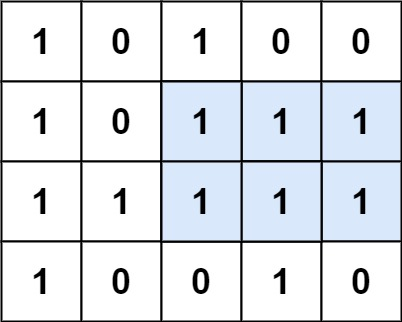85. Maximal Rectangle
Hard
8.6K
139

Given a `rows x cols` binary `matrix` filled with `0`'s and `1`'s, find the largest rectangle containing only `1`'s and return its area.

Example 1:```Input: matrix = [["1","0","1","0","0"],["1","0","1","1","1"],["1","1","1","1","1"],["1","0","0","1","0"]]
Output: 6
Explanation: The maximal rectangle is shown in the above picture.
```

Example 2:

```Input: matrix = [["0"]]
Output: 0
```

Example 3:

```Input: matrix = [["1"]]
Output: 1
```

Constraints:

• `rows == matrix.length`
• `cols == matrix[i].length`
• `1 <= row, cols <= 200`
• `matrix[i][j]` is `'0'` or `'1'`.
Accepted
345.6K
Submissions
768.6K
Acceptance Rate
45.0%

Seen this question in a real interview before?
1/4
Yes
No

Discussion (0)

Related Topics